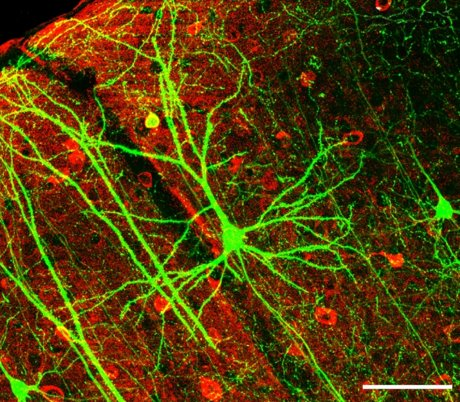# Delay Equations in Neural Fields

Funded by:

UT/EWI

PhD:

Supervisor:

Collaboration:

## Description:

The field of Neuroscience has developed an understanding of the brain on the largest and smallest scales. On the largest scales, brain regions have been mapped to specific functions. On the smallest scales, the major biophysical processes on the cell level have been discovered and modelled. However, brain structures on the intermediate scale are as of yet poorly understood. Neural Field models try to bridge the gap by simulating qualitative behavior of large groups of neurons.

A Neural Field model uses spatial and temporal averaging of the membrane voltage of a population of neurons. Synaptic connections are modelled by a convolution of a connectivity kernel and a non-linear activation function. Delays come into play, as the propagation speed of electric synaptic signals is finite.

We analyze this model in the context of Delay Equations. Using the Functional Analysis of the Sun-Star Calculus it is possible to develop a geometric theory for the qualitative behavior, based on the center manifold reduction and bifurcation analysis.

We investigate a variant of the Neural Field model, which includes diffusion. This models direct electrical connections. For certain functions for the connectivity and delay, we can directly compute the essential spectrum, eigenvalues, eigenvectors and resolvent. This in turn allows us to find parameters for which the system undergoes a Hopf-bifurcation. We can also directly compute the Lyapunov coefficient to determine the stability of the corresponding limit cycle. This allows us to study the effect of diffusion on emerging oscillations.

In the model we assumed the spatial domain to be a (finite) line with Neumann boundary conditions. We are exploring also two-dimensional domains, like a rectangle. We are investigating if it is possible to find similar results for the spectrum and bifurcations in two dimensional domains, including diffusion.

## Output:

Publications